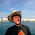## Isomorphic Strings

Given two strings s and t, determine if they are isomorphic.
Two strings are isomorphic if the characters in s can be replaced to get t.
All occurrences of a character must be replaced with another character while preserving the order of characters. No two characters may map to the same character but a character may map to itself.
For example,
Given "egg""add", return true.
Given "foo""bar", return false.
Given "paper""title", return true.

### Analysis:

Using hashmap (dict for python) is a good idea solving this problem, since the two strings require some unique mapping. Note that it also requires no two characters may map to the same char, e.g., s="ab", t = "aa", when we check 'b' in s, although the key 'b' has not been in the map (dict for python), its value 'a' has already been used previously. So, in our implementation, we need to take care of this issue. Here I use two maps to store s->t mapping and t->s mapping, only when both maps has no key/value pairs, new pair is added to maps.

### Code(C++):

class Solution {
public:
bool isIsomorphic(string s, string t) {
map<char,char> mp1;
map<char,char> mp2;
for (int i=0;i<s.size();i++){
if (mp1.find(s[i])== mp1.end() && mp2.find(t[i]) == mp2.end()){
mp1[s[i]] = t[i];
mp2[t[i]] = s[i];
}else{
if (mp1[s[i]]!=t[i] || mp2[t[i]]!=s[i]){
return false;
}
}
}
return true;
}
};


### Code(Python):

class Solution:
# @param {string} s
# @param {string} t
# @return {boolean}
def isIsomorphic(self, s, t):
d1 = {}
d2 = {}
for (cs, ct) in zip(s, t):
if d1.get(cs) is None and d2.get(ct) is None:
d1[cs] = ct
d2[ct] = cs
else:
if d1.get(cs) != ct or d2.get(ct) != cs:
return False
return True


1.1.2.